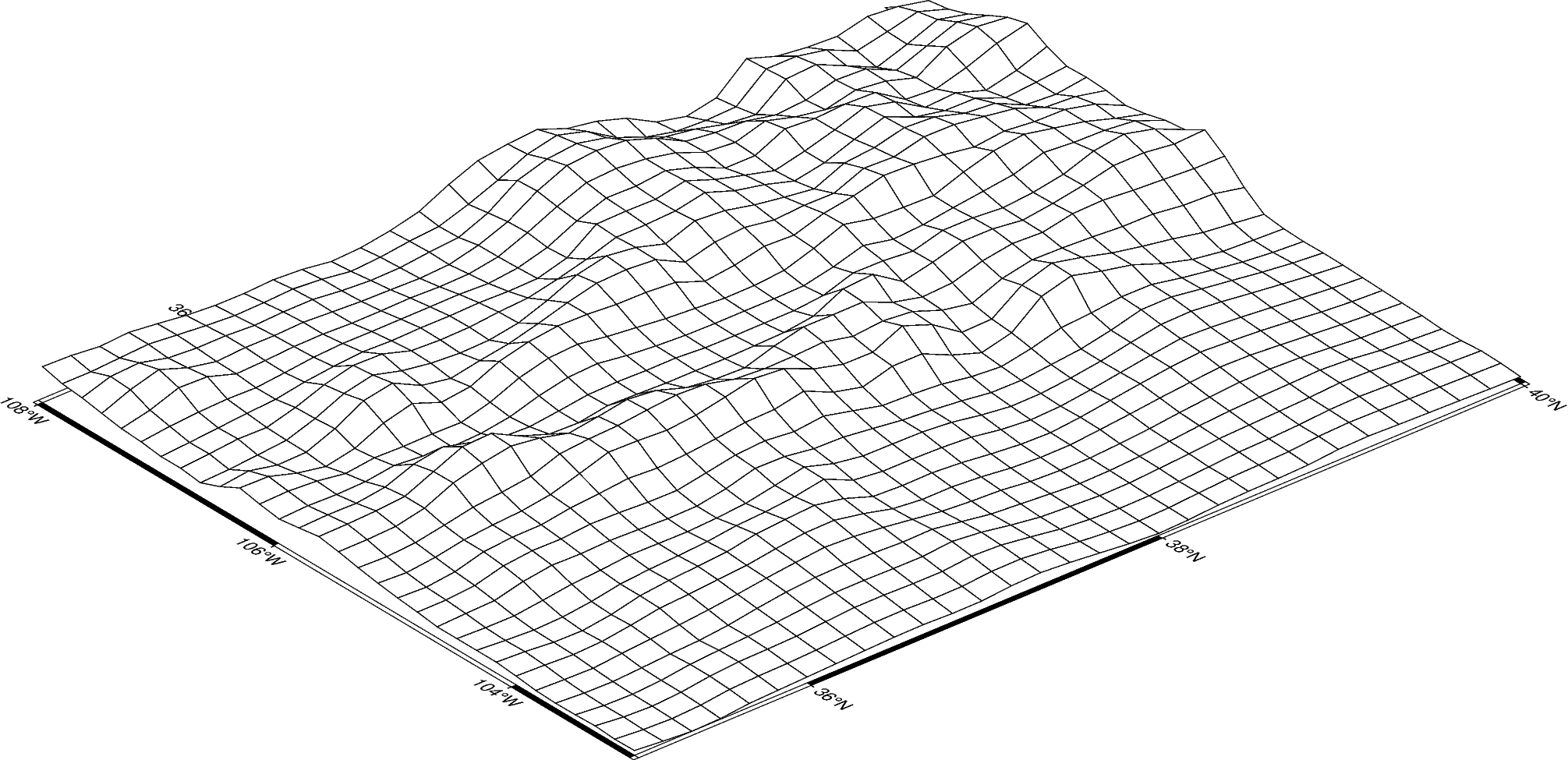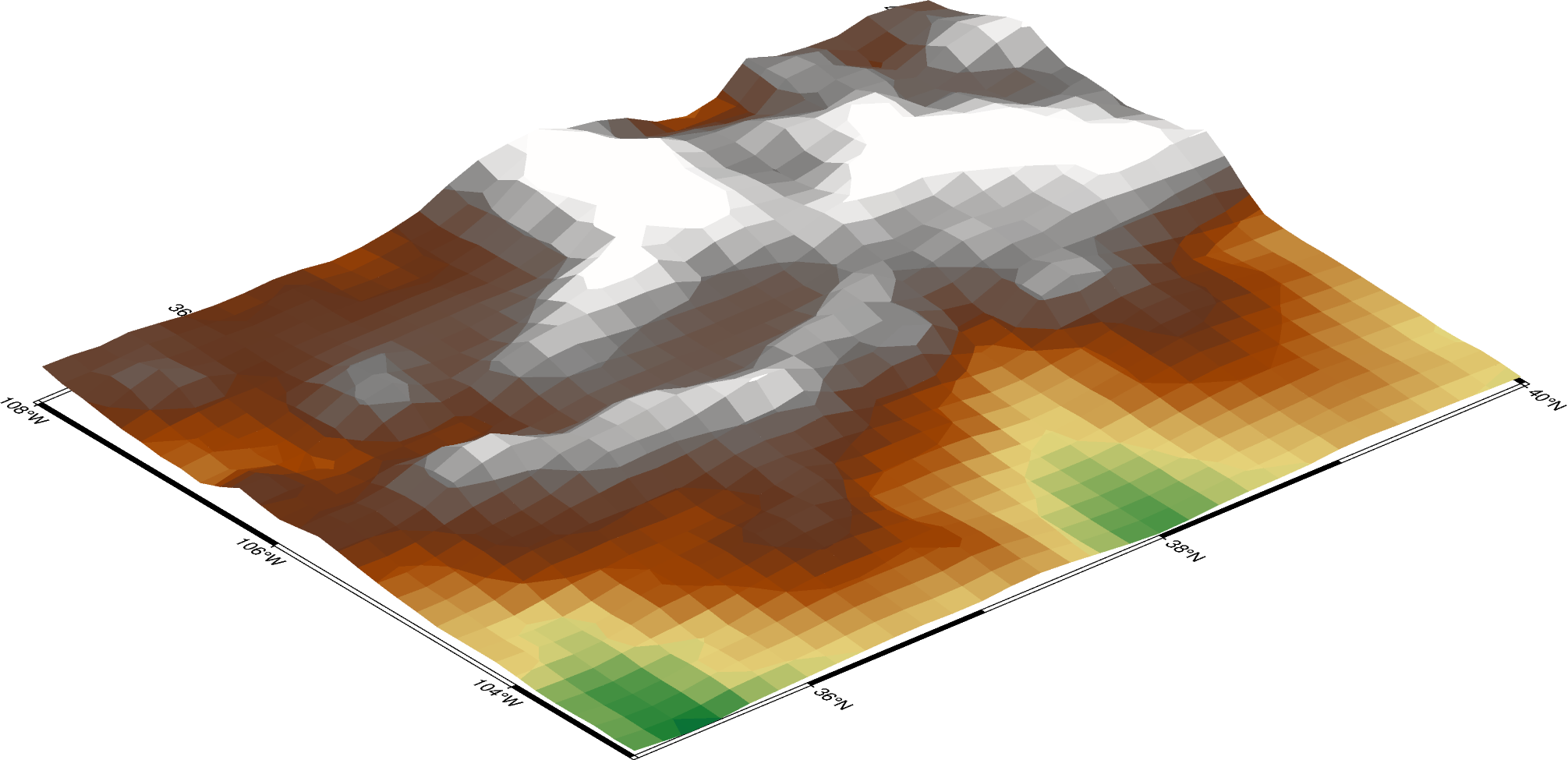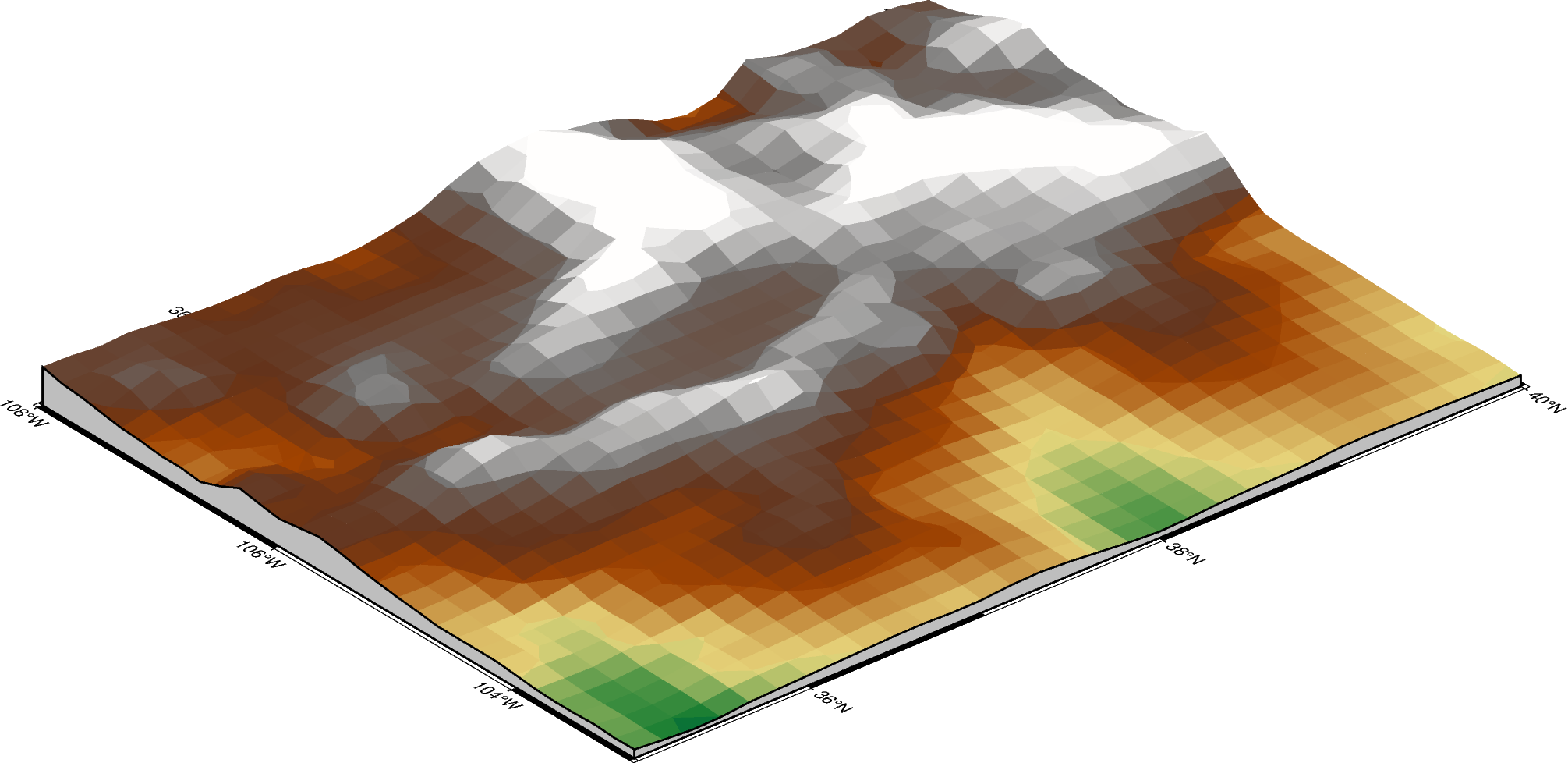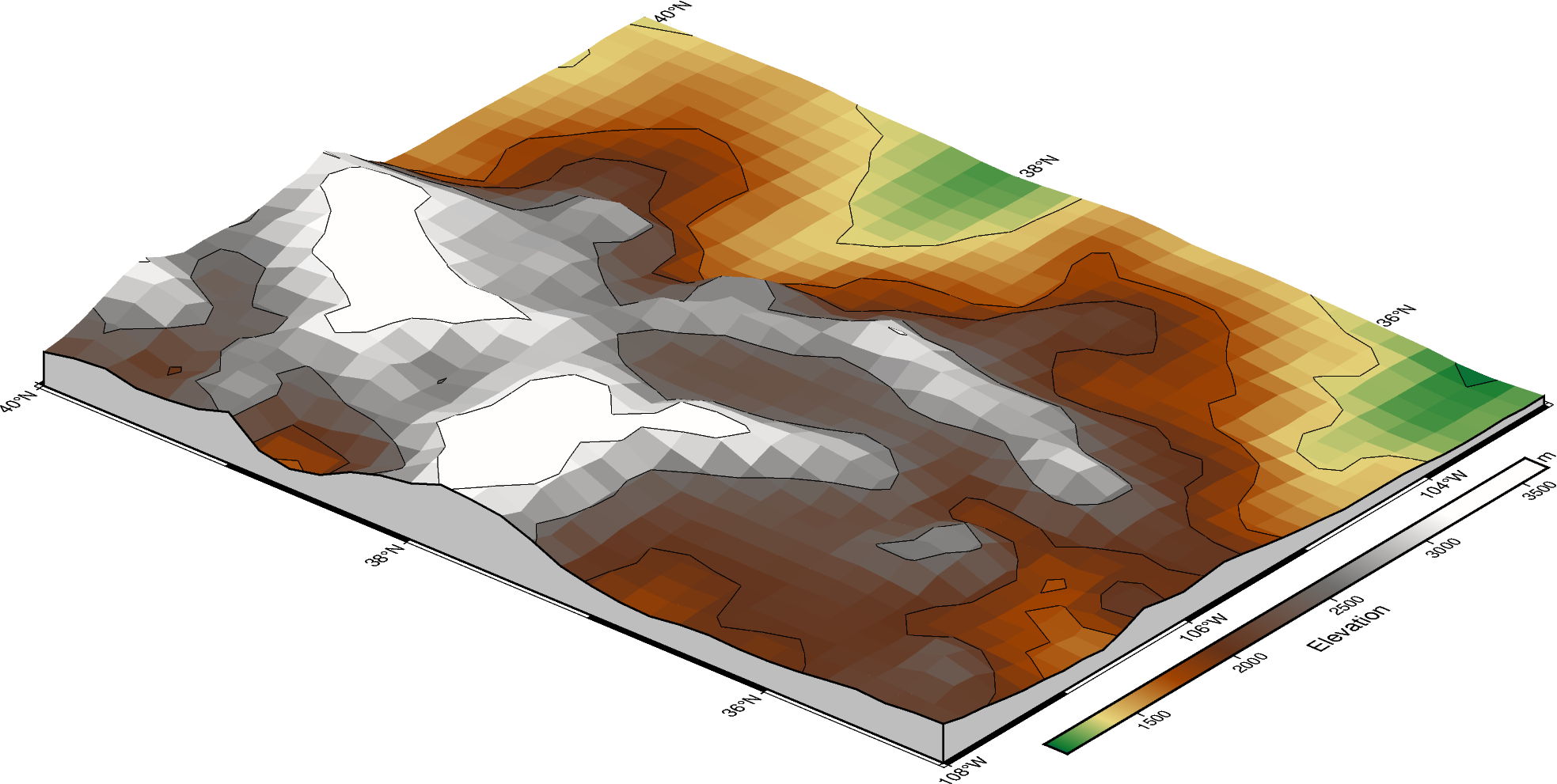# Creating a 3-D perspective image

Create 3-D perspective image or surface mesh from a grid using `pygmt.Figure.grdview`.

```import pygmt

# Load sample earth relief data
grid = pygmt.datasets.load_earth_relief(resolution="10m", region=[-108, -103, 35, 40])
```

The `pygmt.Figure.grdview` method takes the `grid` input. The `perspective` parameter changes the azimuth and elevation of the viewpoint; the default is [180, 90], which is looking directly down on the figure and north is “up”. The `zsize` parameter sets how tall the three-dimensional portion appears.

The default grid surface type is mesh plot.

```fig = pygmt.Figure()
fig.grdview(
grid=grid,
# Sets the view azimuth as 130 degrees, and the view elevation as 30
# degrees
perspective=[130, 30],
# Sets the x- and y-axis labels, and annotates the west, south, and east
# axes
frame=["xa", "ya", "WSnE"],
# Sets a Mercator projection on a 15-centimeter figure
projection="M15c",
# Sets the height of the three-dimensional relief at 1.5 centimeters
zsize="1.5c",
)
fig.show()
```The grid surface type can be set with the `surftype` parameter. The default CPT is turbo and can be customized with the `cmap` parameter.

```fig = pygmt.Figure()
fig.grdview(
grid=grid,
perspective=[130, 30],
frame=["xa", "yaf", "WSnE"],
projection="M15c",
zsize="1.5c",
# Set the surftype to "surface"
surftype="s",
# Set the CPT to "geo"
cmap="geo",
)
fig.show()
```The `plane` parameter sets the elevation and color of a plane that provides a fill below the surface relief.

```fig = pygmt.Figure()
fig.grdview(
grid=grid,
perspective=[130, 30],
frame=["xa", "yaf", "WSnE"],
projection="M15c",
zsize="1.5c",
surftype="s",
cmap="geo",
# Set the plane elevation to 1,000 meters and make the fill "gray"
plane="1000+ggray",
)
fig.show()
```The `perspective` azimuth can be changed to set the direction that is “up” in the figure. The `contourpen` parameter sets the pen used to draw contour lines on the surface. `pygmt.Figure.colorbar` can be used to add a color bar to the figure. The `cmap` parameter does not need to be passed again. To keep the color bar’s alignment similar to the figure, use `True` as the `perspective` parameter.

```fig = pygmt.Figure()
fig.grdview(
grid=grid,
# Set the azimuth to -130 (230) degrees and the elevation to 30 degrees
perspective=[-130, 30],
frame=["xaf", "yaf", "WSnE"],
projection="M15c",
zsize="1.5c",
surftype="s",
cmap="geo",
plane="1000+ggrey",
# Set the contour pen thickness to "0.1p"
contourpen="0.1p",
)
fig.colorbar(perspective=True, frame=["a500", "x+lElevation", "y+lm"])
fig.show()
```Total running time of the script: ( 0 minutes 2.754 seconds)

Gallery generated by Sphinx-Gallery